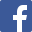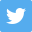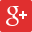1954
Senior Section P W D L F A GD GA Pt Adj Reserve Section Isthmian League `1.` `Walthamstow Avenue` `28` `21` `1` `6` `80` `38` `42` `2.11` `43` `2.` `St. Albans City` `28` `18` `3` `7` `61` `41` `20` `1.49` `39` `3.` `Bromley` `28` `18` `2` `8` `66` `34` `32` `1.94` `38` `4.` `Wycombe Wanderers` `28` `16` `3` `9` `68` `43` `25` `1.58` `35` `5.` `Ilford` `28` `13` `5` `10` `64` `46` `18` `1.39` `31` `6.` `Barking` `28` `15` `1` `12` `55` `51` `4` `1.08` `31` `7.` `Woking` `28` `12` `3` `13` `75` `79` `-4` `0.95` `27` `8.` `Kingstonian` `28` `10` `7` `11` `47` `57` `-10` `0.82` `27` `9.` `Leytonstone` `28` `10` `4` `14` `35` `51` `-16` `0.69` `24` `10.` `Oxford City` `28` `10` `3` `15` `43` `74` `-31` `0.58` `23` `11.` `Clapton` `28` `9` `4` `15` `41` `50` `-9` `0.82` `22` `12.` `Wimbledon` `28` `10` `2` `16` `48` `62` `-14` `0.77` `22` `13.` `Corinthian-Casuals` `28` `9` `3` `16` `50` `65` `-15` `0.77` `21` `14.` `Dulwich Hamlet` `28` `7` `5` `16` `48` `60` `-12` `0.80` `19` `15.` `Romford` `28` `4` `10` `14` `43` `73` `-30` `0.59` `18` ` ` `420` `182` `56` `182` `824` `824` `0` `420` `0` Isthmian League `1.` `Dulwich Hamlet (reserves)` `28` `22` `4` `2` `107` `40` `67` `2.67` `48` `2.` `Wycombe Wanderers (reserves)` `28` `19` `4` `5` `76` `31` `45` `2.45` `42` `3.` `Clapton (reserves)` `28` `17` `2` `9` `60` `41` `19` `1.46` `36` `4.` `Walthamstow Avenue (reserves)` `28` `14` `5` `9` `59` `45` `14` `1.31` `33` `5.` `Bromley (reserves)` `28` `14` `4` `10` `68` `54` `14` `1.26` `32` `6.` `Woking (reserves)` `28` `12` `4` `12` `52` `61` `-9` `0.85` `28` `7.` `Corinthian-Casuals (reserves)` `28` `12` `2` `14` `49` `70` `-21` `0.70` `26` `8.` `Wimbledon (reserves)` `28` `10` `5` `13` `51` `52` `-1` `0.98` `25` `9.` `Leytonstone (reserves)` `28` `10` `5` `13` `54` `58` `-4` `0.93` `25` `10.` `Barking (reserves)` `28` `9` `6` `13` `43` `55` `-12` `0.78` `24` `11.` `Oxford City (reserves)` `28` `10` `4` `14` `48` `66` `-18` `0.73` `24` `12.` `St. Albans City (reserves)` `28` `8` `6` `14` `35` `51` `-16` `0.69` `22` `13.` `Kingstonian (reserves)` `28` `9` `3` `16` `55` `69` `-14` `0.80` `21` `14.` `Romford (reserves)` `28` `6` `6` `16` `43` `79` `-36` `0.54` `18` `15.` `Ilford (reserves)` `28` `7` `2` `19` `43` `71` `-28` `0.61` `16` ` ` `420` `179` `62` `179` `843` `843` `0` `420` `0` `1.` `Kingstonian (A)` `18` `13` `1` `4` `72` `29` `43` `2.48` `27` `2.` `Carshalton Athletic (A)` `18` `13` `4` `1` `83` `39` `44` `2.13` `30` `3.` `Wimbledon (A)` `18` `11` `4` `3` `65` `30` `35` `2.17` `26` `4.` `Woking (A)` `18` `10` `2` `6` `68` `43` `25` `1.58` `22` `5.` `Sutton United (A)` `17` `8` `3` `6` `45` `33` `12` `1.36` `19` `7.` `Epsom (A)` `16` `8` `3` `5` `39` `38` `1` `1.03` `19` `7.` `Banstead Athletic (A)` `18` `8` `2` `8` `62` `48` `14` `1.29` `18` `8.` `Molesey (A)` `18` `4` `1` `13` `22` `83` `-61` `0.27` `9` `9.` `Walton & Hersham (A)` `17` `2` `3` `12` `31` `69` `-38` `0.45` `7` `10.` `Vickers (A)` `16` `0` `0` `16` `16` `84` `-68` `0.19` `0` `11.` `Chertsey Town (A)` `0` `0` `0` `0` `0` `0` `0` `0.00` `0` ` ` `174` `77` `23` `74` `503` `496` `7` `177` `0` Incorrect."Final" table, some matches not played.Chersey "A" withdrew in the new year and their record was expunged.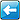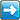This page has been visited 641 times. The contents of this site are (c) their respective owners. If you wish to use anything within, please check their status first. If you have any information, photographs, comments, additions, memorabilia or corrections to this site please contact us